##### Quantum Physics For Dummies, Revised EditionYou can determine what happens to the wave function when you swap particles in a multi-particle atom. Whether the wave function is symmetric or antisymmetric under such operations gives you insight into whether two particles can occupy the same quantum state.

Given that Pij2 = 1, note that if a wave function is an eigenfunction of Pij, then the possible eigenvalues are 1 and –1. That is, for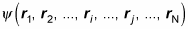an eigenfunction of Pij looks like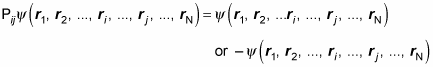That means there are two kinds of eigenfunctions of the exchange operator: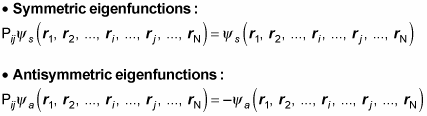Now take a look at some symmetric and some antisymmetric eigenfunctions. How about this one — is it symmetric or antisymmetric?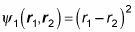You can apply the exchange operator P12: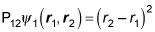Note that because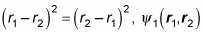is a symmetric wave function; that’s because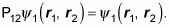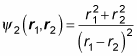Again, apply the exchange operator, P12: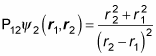Okay, but because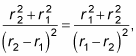you know that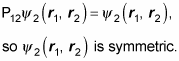Here’s another one: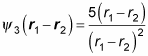Now apply P12: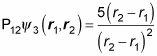How does that equation compare to the original one? Well,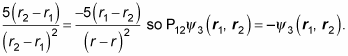Therefore,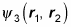is antisymmetric.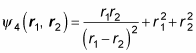To find out, apply P12: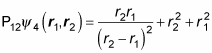All right — how’s this compare with the original equation?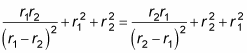Okay —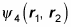is symmetric.

You may think you have this process down pretty well, but what about this next wave function?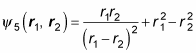Start by applying P12: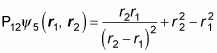So how do these two equations compare?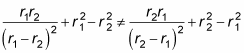That is,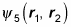is neither symmetric nor antisymmetric. In other words,is not an eigenfunction of the P12 exchange operator.